CAT  >  Test: Pipes And Cistern- 1

# Test: Pipes And Cistern- 1

Test Description

## 10 Questions MCQ Test Quantitative Aptitude (Quant) | Test: Pipes And Cistern- 1

Test: Pipes And Cistern- 1 for CAT 2022 is part of Quantitative Aptitude (Quant) preparation. The Test: Pipes And Cistern- 1 questions and answers have been prepared according to the CAT exam syllabus.The Test: Pipes And Cistern- 1 MCQs are made for CAT 2022 Exam. Find important definitions, questions, notes, meanings, examples, exercises, MCQs and online tests for Test: Pipes And Cistern- 1 below.
Solutions of Test: Pipes And Cistern- 1 questions in English are available as part of our Quantitative Aptitude (Quant) for CAT & Test: Pipes And Cistern- 1 solutions in Hindi for Quantitative Aptitude (Quant) course. Download more important topics, notes, lectures and mock test series for CAT Exam by signing up for free. Attempt Test: Pipes And Cistern- 1 | 10 questions in 10 minutes | Mock test for CAT preparation | Free important questions MCQ to study Quantitative Aptitude (Quant) for CAT Exam | Download free PDF with solutions
 1 Crore+ students have signed up on EduRev. Have you?
Test: Pipes And Cistern- 1 - Question 1

### One pipe can fill a tank four times as fast as another pipe. If together the two pipes can fill the tank in 36 minutes, then the slower pipe alone will be able to fill the tank in:

Detailed Solution for Test: Pipes And Cistern- 1 - Question 1

Suppose the slower pipe can fill the tank in x minutes.
Then the faster pipe can fill in x/4 minutes.
Part filled by the slower pipe in 1 min =1/x
Part filled by the faster pipe in 1 min =4/x
Part filled by the both the pipes in 1 min =1/x +4/x

Therefore, both the pipes can fill together in 36 minutes.
Part filled by both in 1 minute = 1/36.
1/x + 4/x = 1/36
5/x = 1/36
x = 5 * 36 = 180

Test: Pipes And Cistern- 1 - Question 2

### Three taps A, B and C can fill a tank in 12, 15 and 20 hours respectively. if A is open all the time and B and C are open for one hour each alternately, the tank will be full in: A.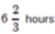B. 6 hours  C.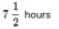D. 7 hours

Detailed Solution for Test: Pipes And Cistern- 1 - Question 2

(A + B)'s 1 hour's work =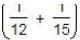= 9/60 = 3/20.
(A+C)'s hour's work =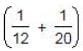= 8/60 = 2/15.
Part filled in 2 hrs =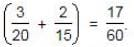Part filled in 6 hrs =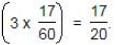Remaining part =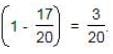Now, it is the turn of A and B and 3/20 part is filled by A and B in 1 hour.
∴ Total time taken to fill the tank = (6+1) hrs = 7 hrs

Test: Pipes And Cistern- 1 - Question 3

### A leak in the bottom of a tank can empty the full tank in 6 hours. An inlet pipe fills water at the rate of 4 liters a minute. When the tank is full, the inlet is opened and due to the leak, the tank is empty in 24 hours. How many liters does the cistern hold?

Detailed Solution for Test: Pipes And Cistern- 1 - Question 3

Part emptied by the leak in 1 hour = 1/6
Net part emptied by the leak and the inlet pipe in 1 hour = 1/24
Part filled by the inlet pipe in 1 hour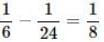i.e., inlet pipe fills the tank in 8 hours = (8 * 60) minutes = 480 minutes
Given that the inlet fills water at the rate of 4 liters a minute
Hence, water filled in 480 minutes = 480 * 4 = 1920 litre
i.e., The cistern can hold 1920 litre

Test: Pipes And Cistern- 1 - Question 4

A cistern can be filled by a tap in 3 hours while it can be emptied by another tap in 8 hours. If both the taps are opened simultaneously, then after how much time will the cistern get filled?

Detailed Solution for Test: Pipes And Cistern- 1 - Question 4

Part filled by first tap in 1 hour = 1/3
Part emptied by second tap 1 hour = 1/8
Net part filled by both these taps in
1 hour
= 1/3 − 1/8 = 5/24
i.e, the cistern gets filled in 24/5 hours
= 4.8 hours.

Test: Pipes And Cistern- 1 - Question 5

Three pipes A, B and C can fill a tank in 6 hours. After working at it together for 2 hours, C is closed and A and B can fill the remaining part in 7 hours. The number of hours taken by C alone to fill the tank is:

Detailed Solution for Test: Pipes And Cistern- 1 - Question 5

part filled in 2 hours =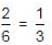Remaining  part =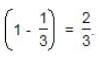∴ (A + B)'s 7 hour's work = 2/3
(A + B)'s 1 hour's work = 2/21
∴ C's 1 hour's work = {(A + B + C)'s 1 hour's  work} - {(A + B)'s 1 hour's work}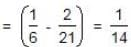∴ C alone can fill the tank in 14 hours.

Test: Pipes And Cistern- 1 - Question 6

A large tanker can be filled by two pipes A and B in 60 minutes and 40 minutes respectively. How many minutes will it take to fill the tanker from empty state if B is used for half the time and A and B fill it together for the other half?

Detailed Solution for Test: Pipes And Cistern- 1 - Question 6

Part filled by (A + B) in 1 minute =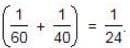Suppose the tank is filled in x minutes.
Then,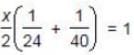⇒ x = 30 min.

Test: Pipes And Cistern- 1 - Question 7

A tap can fill a tank in 6 hours. After half the tank is filled, three more similar taps are opened. What is the total time taken to fill the tank completely?

Detailed Solution for Test: Pipes And Cistern- 1 - Question 7

Time taken by one tap to fill half of the tank = 3 hrs.
Part filled by the four taps in 1 hour =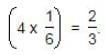Remaining part =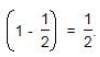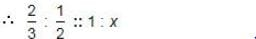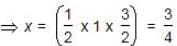hours i.e, 45 mins.
So, total time taken = 3 hrs. 45 mins.

Test: Pipes And Cistern- 1 - Question 8

Two taps A and B can fill a tank in 5 hours and 20 hours respectively. If both the taps are open then due to a leakage, it took 40 minutes more to fill the tank. If the tank is full, how long will it take for the leakage alone to empty the tank?

Detailed Solution for Test: Pipes And Cistern- 1 - Question 8

Part filled by pipe A in 1 hour = 1/5
Part filled by pipe B in 1 hour = 1/20
Part filled by pipe A and B in 1 hour =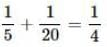i.e., A and b together can fill the tank in 4 hours
Given that due to the leakage, it took 40 minutes more to fill the tank.
i.e., due to the leakage, the tank got filled in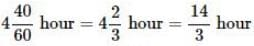⇒ Net part filled by pipe A and B and the leak in 1 hour = 3/14
⇒ Part emptied by the leak in 1 hour =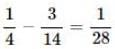i.e., the leak can empty the tank in 28 hours.

Test: Pipes And Cistern- 1 - Question 9

A tap can fill a tank in 4 hours. After half the tank is filled, two more similar taps are opened. What is the total time taken to fill the tank completely?

Detailed Solution for Test: Pipes And Cistern- 1 - Question 9

A tap can fill a tank in 4 hours.
Therefore the tap can fill half the tank in 2 hours.
Remaining part = 1/2
After half the tank is filled, two more similar taps are opened.
Hence, total number of taps becomes 3.
Part filled by one tap in 1 hour = 1/4
Part filled by three taps in 1 hour
= 3 × 1/4 = 3/4
i.e., 4 taps can fill remaining half in 40 minutes.
Total time taken = 2 hour + 40 minute
= 2 hour 40 minutes

Test: Pipes And Cistern- 1 - Question 10

Bucket P has thrice the capacity as bucket Q. It takes 80 turns for bucket P to fill the empty drum. How many turns it will take for both the buckets P and Q, having each turn together to fill the empty drum?

Detailed Solution for Test: Pipes And Cistern- 1 - Question 10

Let capacity of bucket P = x
Then capacity of bucket Q =  x/3
Given that it takes 80 turns for bucket P  to fill the empty drum
⇒ capacity of the drum = 80x
Number of turns required if both P and Q are used =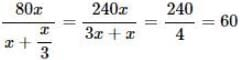## Quantitative Aptitude (Quant)

163 videos|152 docs|131 tests
 Use Code STAYHOME200 and get INR 200 additional OFF Use Coupon Code
Information about Test: Pipes And Cistern- 1 Page
In this test you can find the Exam questions for Test: Pipes And Cistern- 1 solved & explained in the simplest way possible. Besides giving Questions and answers for Test: Pipes And Cistern- 1, EduRev gives you an ample number of Online tests for practice

## Quantitative Aptitude (Quant)

163 videos|152 docs|131 tests

### How to Prepare for CAT

Read our guide to prepare for CAT which is created by Toppers & the best Teachers